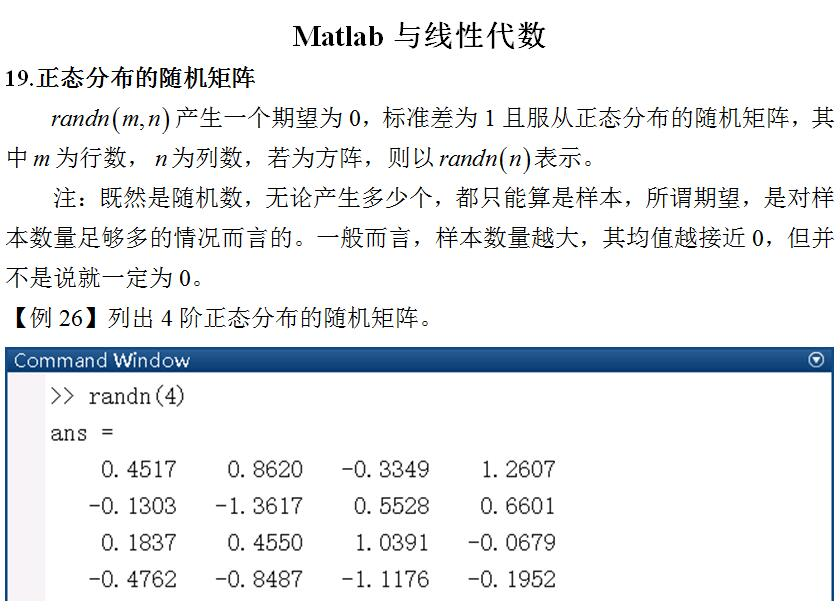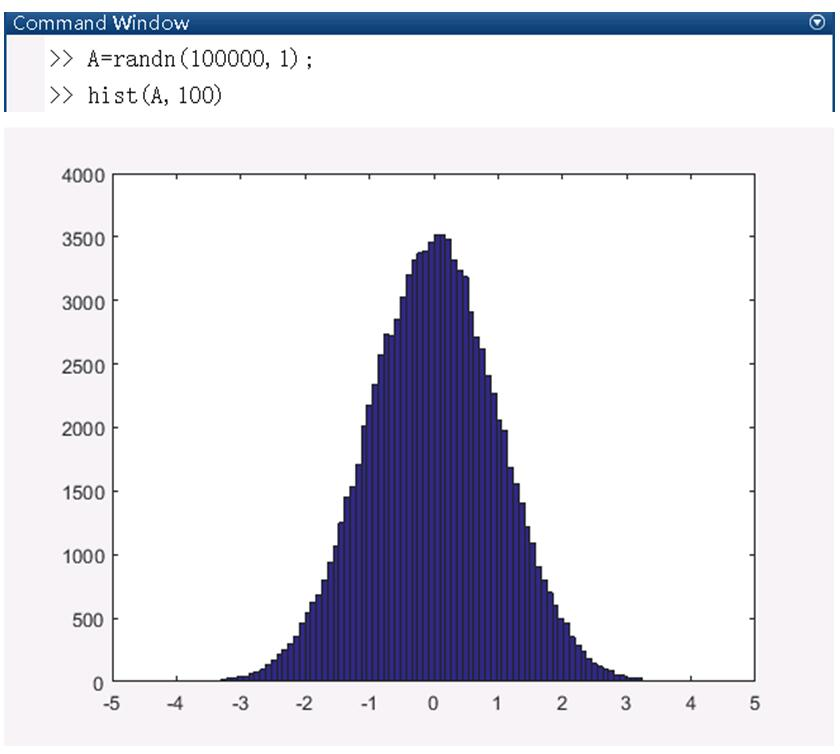• matlab正态分布随机矩阵
千次阅读
2020-02-15 11:42:02

### 1、表达式

fix(a+(b-a+1)*x)：产生[a，b]区间上均匀分布的随机整数或者矩阵。x使用rand函数产生。详见代码示例。

m + nx：产生均值为m、方差为n平方的随机数或者矩阵。x使用randn函数产生。详见代码示例。

### 2、代码示例

clc;
clear all;

D = fix(10 + (99-10+1)*rand(1)) %产生随机两位数，[10-99]
E = 10 + 2*randn(2)     %产生均值为10、方差为4的矩阵

运行结果：

D =

43

E =

11.1056    7.7647
12.0782   12.5213

更多相关内容
• 本代码主要利用MATLAB工具实现MATLAB——创建标准正态分布随机矩阵，简单明了，易于理解
• 本资源有两段代码，一段是用rand随机数循环算pi的值和矩阵算pi的值并进行对比和耗时...另一段是用matlab内置伪随机算法rand生成正态分布概率密度函数，并包含图像的生成和对比。有问题可以私信，有问必答，欢迎你的购买
• 功能：生成服从正态分布的随机数 语法： R＝normrnd(MU,SIGMA) R＝normrnd(MU,SIGMA,m) R＝normrnd(MU,SIGMA,m,n) 说 明： R＝normrnd(MU,SIGMA)：生成服从正态分布(MU参数代表均值，DELTA参数代表标准差)的随机数...

功能：生成服从正态分布的随机数
语法：
R＝normrnd(MU,SIGMA)
R＝normrnd(MU,SIGMA,m)
R＝normrnd(MU,SIGMA,m,n)

说 明：
R＝normrnd(MU,SIGMA)：生成服从正态分布(MU参数代表均值，DELTA参数代表标准差)的随机数。输入的向量或矩阵MU和SIGMA必须形式相同，输出R也和它们形式相同。标量输入将被扩展成和其它输入具有 相同维数的矩阵。

R＝norrmrnd(MU,SIGMA,m)：生成服从正态分布(MU参数代表均值，DELTA参数代表标准差)的 随机数矩阵，矩阵的形式由m定义。m是一个1×2向量，其中的两个元素分别代表返回值R中行与列的维数。

R＝normrnd(MU,SIGMA,m,n)： 生成m×n形式的正态分布的随机数矩阵。

例：生成正态分布随机数。
>> a=normrnd(0,1)

a =

-1.4814

>> a=normrnd(0,1,1,6)

a =

1.1287   -0.2900    1.2616    0.4754    1.1741    0.1269

>> a=normrnd(0,1,[1 6])

a =

0.1555    0.8186   -0.2926   -0.5408   -0.3086   -1.0966

>> a=normrnd(10,2,2,3)

a =

13.6280   13.6090   11.0531
10.6240    8.5538    9.4795

展开全文matlab 高斯分布
• 本图文介绍了如何利用Matlab实现正态分布随机矩阵

本图文介绍了如何利用Matlab实现正态分布的随机矩阵。展开全文matlab
• 文章目录代码mvnrnd输入参数mu——多元正态分布的均值sigma——多元正态分布的协方差n——多元随机数的个数mvnrnd输出参数R——多元正态随机数 代码 生成指定均值向量为(3, 2)，协方差矩阵为(11.51.54)\left(\begin...

# 代码

生成指定均值向量为(3, 2)，协方差矩阵为 ( 1 1.5 1.5 4 ) \left(\begin{aligned}&1&1.5\\&1.5&4\end{aligned}\right) 的二元正态分布的随机数：

mu = [3 2]; % 均指向量
nov = [1 1.5; 1.5 4]; % 协方差矩阵

% 生成100个二元正态分布随机数
R = mvnrnd(mu, nov, 100);

% 绘制二元正态分布散点图
scatter(R(:,1),R(:,2),'filled');
grid on;# mvnrnd输入参数

## mu——多元正态分布的均值

：数值向量 | 数值矩阵

含义：多元正态分布的平均值，指定为 1×d数值向量m×d数值矩阵。如果 mu 是一个向量，那么 mvnrd 复制该向量以匹配 sigma 的后续维度。如果 mu 是一个矩阵，那么 mu 的每一行就是单个多元正态分布的均值向量。

数据类型：整型 | 双精度浮点型

## sigma——多元正态分布的协方差

：对称半正定矩阵 | 数字数组

含义：多元正态分布的协方差，指定为 d×d对称半正定矩阵d×d×m 数值数组。如果 sigma 是一个矩阵，那么 mvnrnd 复制该矩阵以匹配 mu 中的行数。如果 sigma 是一个数组，那么 sigma 的每一个元素，sigma(: , :, i) 是单个多元正态分布的协方差矩阵，因此是一个对称的半正定矩阵。

如果协方差矩阵是对角矩阵，包含沿对角线的方差和零协方差，则还可以将 sigma 指定为 1×d向量 或 仅包含对角线项的1×d×m数组

数据类型：整型 | 双精度浮点型

## n——多元随机数的个数

：正标量整数

含义：多变量随机数的数目，指定为正标量整数。n 指定 R 中的行数。

数据类型：整型 | 双精度浮点型

# mvnrnd输出参数

## R——多元正态随机数

：数值矩阵

多元正态随机数，返回为以下值之一：

• mxd数值矩阵，其中 m 和 d 是由 μ 和 σ 指定的尺寸
• n×d数值矩阵，其中 n 是指定的输入参数，d 是由 mu 和 sigma 指定的维数

如果 mu 是矩阵，sigma 是数组，那么 mvnrnd 使用 mu(i, : ) 和 sigma(:, :, i) 计算 R(i, : )。

展开全文matlab
• normrnd_normfit 帮助用户生成一个正态分布随机集数据，然后在数据的顶部和后面拟合高斯曲线计算其均值和标准差。 它可以帮助用户检查 NORMRND 函数完成了它的工作。它绘制了原始直方图和拟合的直方图。 normrnd_...matlab
• ## matlab生成正态分布

千次阅读 2022-05-24 17:00:42
randn 产生标准正态分布 N(0,1) 如果你想生成均值为a，方差为b的非标准正态分布N(a,b)，则为：a+b*randn(m,n)。其中：m为行数，n为列数。...产生对数正态分布随机数 mvnrnd 产生多元正态分布随机数 ...matlab
• 01首先，我们需要将我们要分析的数据文件整理为矩阵文件，即行列分明的数据文件。02我们打开matlab之后，点击菜单栏里的“importdata”,准备加载我们需要统计分析的数据。03打开加载界面之后，我们找到我们要加载的...
• 产生一组正态分布N（μ,σ^2）的高斯随机数，其样本个数为N，估计该序列的均值、方差和相关函数。（1）互相关函数xcorr用法c=xcorr(x,y)；C=xcorr(x)a=normrnd(1,2,1,1000);c=xcorr(x)%计算自相关函数。title('自...matlab
• 本博文源于matlab基础，主要对协方差矩阵如何生成...例子：求出5*6阶服从正态分布随机数的协方差矩阵 >> p = randn(5,6) p = 0.6630 0.4853 1.5352 0.0359 -2.0543 -0.0787 -0.8542 -0.5955 -0.6065 -0.6275matlab
• Date: 2018.8.5 转载自：... 功能：生成服从正态分布的随机数 语法： R＝normrnd(MU,SIGMA) R＝normrnd(MU,SIGMA,m) R＝normrnd(MU,SIGMA,m,n) 说 明： R＝normrnd(MU,SIG...
• 本博文源于matlab概率论内容。主要讲述正态分布的随机数绘制matlab
• MATLAB如何使用normrnd函数生成正态分布随机数【语法说明】R=normrnd(mu,sigma)：生成服从参数为mu和sigma的正态分布的随机数。mu为均值，sigma为标准差。R是与mu、sigma同型的数组，如果mu和sigma之一为标量，则该...
• matlab中，很容易生成具有均值和标准差的正态分布随机向量。 从帮助兰德：从均值1和标准的正态分布生成值偏差2。r = 1 + 2. * randn(100,1);现在我有一个协方差矩阵C，我想生成N(0，C)。但是我该怎么办呢？从randn...
• 其中 为产生矩阵的行数和列数， 为产生矩阵的个数，产生的矩阵中的每个元素均为服从 N(0,1)N(0, 1)N(0,1) 的标准正态分布的元素。使用如下命令产生一个 的服从的随机数矩阵： 绘制图像效果如下： 可以看到这里随机数...matlab
• %产生M行N列的随机数矩阵N=8;miu1=1;%第一个分布的参数sigma1=2;%第一个分布的参数miu2=6;%第二个分布的参数sigma2=1;%第二个分布的参数R = 0.2*normrnd(miu1,sigma1,M,N)+0.8*normrnd(miu2,sigma2,M,N);单点的概率...
• 利用matlab产生正态分布数组 思路 课本 2.6 节——“数字噪声的产生”中介绍了两种产生标准正态分布随机数列的方法： 1) 利用随机数字生成器产生 12 个 0~1 之间均匀分布的随机数，通过对这 12 个随机数字 求和来...
• https://reborn.blog.csdn.net/article/details/82855090 https://blog.csdn.net/guziwen/article/details/100049541 https://blog.csdn.net/s334wuchunfangi/article/details/8163551
• 2.randn函数——例：R=randn 返回一个从标准正态分布中得到的随机标量。 （以上两种函数的用法很多，详细的可以去MATLAB “help”中获取。） （想获得具体多少个数据，在上述函数后加一些数字便可。具体用法看...matlab
• R =mvnrnd(MU,SIGMA)——从均值为MU，协方差为SIGMA的正态分布中抽取n*d的矩阵R(n代表抽取的个数，d代表分布的维数)。MU为n*d的矩阵，R中的每一行为以MU中对应的行为均值的正态分布中抽取的一个样本。SIGMA为d*d的...
• X = rmvnrnd(MU,SIG,N,A,B) 在 N×P 矩阵 X a 中返回从 P 维多元正态中抽取的随机样本均值 MU 和协方差 SIG 截断为 a 的分布由不等式 Ax<=B 定义的超平面界定的区域。 [X,RHO,NAR,NGIBBS] = rmvnrnd(MU,SIG,N,A,B...matlab
• randMat：生成在指定区间 [a,b] 上具有均匀或正态分布的随机数矩阵，或者对于任意数量的 a,b 对具有特定的均值 (a) 和方差 (b)。 对于均匀分布，MATLAB 函数 rand() 返回的值乘以 (ba)，然后添加到 a。 对于正态分布...matlab
• 极大似然估计详解下面用MATLAB实现正态分布的ML估计% 二维正态分布的两分类问题 (ML估计)clc;clear;% 两个类别数据的均值向量Mu = [0 0; 3 3]';% 协方差矩阵S1 = 0.8 * eye(2);S(:, :, 1) = S1;S(:, :, 2) = S1;% ...
• 1.语法 X = randn X = randn(n) X = randn(sz1,…,szN) X = randn(sz) ...X = randn 返回一个从标准正态分布中得到的随机标量。 X = randn(n) 返回由正态分布的随机数组成的 n×n 矩阵。 X = randn(sz1,…,s...matlab
• MATLAB如何使用normstat函数计算正态分布的期望与方差【语法说明】[M,V]=normstat(mu,sigma)：给出正态分布的参数 mu 与sigma，求其期望与方差。M、V是与mu和sigma同型的数组，如果输入参数其中之一为标量，则该参数...
• 在FEX上有许多解决相同问题的实现，我对使用它们不是很有信心，因为它们有时无法收敛（使用fsolve等），或者会产生警告，提示协方差矩阵接近奇异。 这是一种简单的蛮力方法，可以记录接受的样本，调整试验大小并重复...matlab...